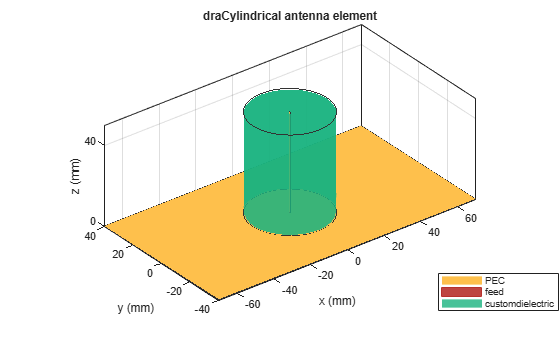draCylindrical

Create cylindrical dielectric resonator antenna

Description

The draCylindrical object creates a cylindrical dielectric resonator antenna. The cylindrical dielectric resonator antenna consists of a cylindrical dielectric placed on the ground plane. It has high power-handling capacity and can provide high gain and bandwidth. These antennas are more suitable for use at microwave frequencies. Cylindrical dielectric resonator antennas are widely used in medium- and long-range communications.Creation

Description

example

ant = draCylindrical creates a cylindrical dielectric resonator antenna with dimensions for a resonant frequency of 1.5 GHz. The default antenna is probe fed with the feedpoint at the origin.

example

ant = draCylindrical(Name,Value) sets Properties using one or more name-value pairs. For example, draCylindrical('ResonatorRadius',0.04) creates a cylindrical dielectric resonator antenna with the radius of the dielectric resonator set to 40 mm.

Properties

expand all

Radius of the dielectric resonator, specified as a positive scalar in meters.

Data Types: double

Type of dielectric material used as a substrate, specified as a dielectric material object. You can choose any material from the DielectricCatalog or use your own dielectric material. For more information, see dielectric. For more information on dielectric substrate meshing, see Meshing.

Note

The substrate dimensions must be lesser than the ground plane dimensions.

Example: d = dielectric('FR4'); 'Substrate',d

Example: d = dielectric; d.Name = 'sub1'; d.EpsilonR = 2.3; d.LossTangent = 0.002; d.Thickness = 0.01; ant.Substrate = d;

Ground plane length, specified as a positive scalar in meters. By default, ground plane length is measured along the x-axis. Set 'GroundPlaneLength' to Inf to use the infinite ground plane technique for antenna analysis.

Example: 'GroundPlaneLength',120e-3

Data Types: double

Ground plane width, specified as a positive scalar in meters. By default, ground plane width is measured along the y-axis. Set 'GroundPlaneWidth' to Inf to use the infinite ground plane technique for antenna analysis.

Example: 'GroundPlaneWidth',118e-3

Data Types: double

Width of the feed, specified as a positive scalar in meters.

Example: 'FeedWidth',5e-05

Data Types: double

Height of the feed, specified as a positive scalar in meters.

Example: 'FeedHeight',0.060

Data Types: double

Signed distance of the feedpoint from the center of the ground plane, specified as a two-element vector in meters.

Example: 'FeedOffset',[–0.0070 0.01]

Data Types: double

Type of the metal used as a conductor, specified as a metal material object. You can choose any metal from the MetalCatalog or specify a metal of your choice. For more information, see metal. For more information on metal conductor meshing, see Meshing.

Example: m = metal('Copper'); 'Conductor',m

Example: m = metal('Copper'); ant.Conductor = m

Tilt angle of the antenna, specified as a scalar or vector with each element unit in degrees. For more information, see Rotate Antennas and Arrays.

Example: 'Tilt',90

Example: ant.Tilt = 90

Example: 'Tilt',[90 90],'TiltAxis',[0 1 0;0 1 1] tilts the antenna at 90 degrees about the two axes defined by the vectors.

Data Types: double

Tilt axis of the antenna, specified as:

• Three-element vector of Cartesian coordinates in meters. In this case, each coordinate in the vector starts at the origin and lies along the specified points on the X-, Y-, and Z-axes.

• Two points in space, each specified as three-element vectors of Cartesian coordinates. In this case, the antenna rotates around the line joining the two points in space.

• A string input describing simple rotations around one of the principal axes, 'X', 'Y', or 'Z'.

Example: 'TiltAxis',[0 1 0]

Example: 'TiltAxis',[0 0 0;0 1 0]

Example: ant.TiltAxis = 'Z'

Lumped elements added to the antenna feed, specified as a lumpedElement object handle. You can add a load anywhere on the surface of the antenna. By default, the load is at the feed. For more information, see lumpedElement.

Object Functions

 show Display antenna or array structure; display shape as filled patch axialRatio Axial ratio of antenna beamwidth Beamwidth of antenna charge Charge distribution on metal or dielectric antenna or array surface current Current distribution on metal or dielectric antenna or array surface design Design prototype antenna or arrays for resonance around specified frequency efficiency Radiation efficiency of antenna EHfields Electric and magnetic fields of antennas; Embedded electric and magnetic fields of antenna element in arrays impedance Input impedance of antenna; scan impedance of array mesh Mesh properties of metal or dielectric antenna or array structure meshconfig Change mesh mode of antenna structure optimize Optimize antenna or array using SADEA optimizer pattern Radiation pattern and phase of antenna or array; Embedded pattern of antenna element in array patternAzimuth Azimuth pattern of antenna or array patternElevation Elevation pattern of antenna or array rcs Calculate and plot radar cross section (RCS) of platform, antenna, or array returnLoss Return loss of antenna; scan return loss of array sparameters Calculate S-parameter for antenna and antenna array objects vswr Voltage standing wave ratio of antenna

Examples

collapse all

Create a cylindrical dielectric resonator antenna with default properties.

ant = draCylindrical
ant =
draCylindrical with properties:

Substrate: [1x1 dielectric]
GroundPlaneLength: 0.1400
GroundPlaneWidth: 0.0800
FeedWidth: 1.0000e-03
FeedHeight: 0.0500
FeedOffset: [0 0]
Conductor: [1x1 metal]
Tilt: 0
TiltAxis: [1 0 0]

View the antenna using the show function.

show(ant)Plot the radiation pattern of the cylindrical dielectric resonator antenna at a frequency of 4 GHz.

pattern(ant,4e9)Create a cylindrical dielectric resonator antenna with FR4, Teflon, and foam as substrates.

ant = draCylindrical;
d = dielectric('FR4','Teflon','Foam');
d.Thickness = [ant.Substrate.Thickness/3 ant.Substrate.Thickness/3 ant.Substrate.Thickness/3];
ant.Substrate = d;
ant = draCylindrical('Substrate',d);
show(ant)Create a cylindrical dielectric resonator antenna with substrates having relative permittivity as 2.3 and 4.5, respectively. The value of loss tangent for both the substrates is 0.002.

ant = draCylindrical;
d = dielectric;
d.Name = {'sub1','sub2'};
d.EpsilonR = [2.3 4.5];
d.LossTangent = [0.002 0.002];
d.Thickness = [ant.Substrate.Thickness/2 ant.Substrate.Thickness/2];
ant.Substrate = d;
show(ant)